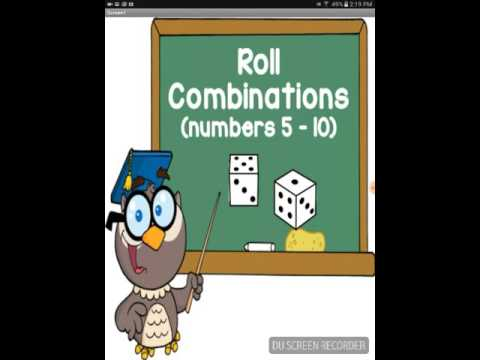# Roll Combinations (numbers 5-10) Game App 1.0 For Android APK Download

## Description of Roll Combinations (numbers 5-10) Game App 1.0 For Android APK Download

Roll Combinations (numbers 5-10) is an app that will help your studentsâ practice subitizing and addition at the same time. Subitizing is being able to instantly recognize how many dots there are in a group. Subitizing helps students form mental pictures of numbers and realize that a number can be represented in many ways. This app provides one player games or two player games for combinations of numbers between 5 and 10. This is a perfect app for your students to âwarm upâ their brain before your math lessons or during math centres. Students will practice adding up the rolled dice up to 10.

How it works:

Roll Combinations of 5 (one player) & Roll Combinations of 5 (two players)
This game includes random dice being ârolledâ which include 0and1, 0and2, 0and3, 0and4, 0and5, 1and1, 1and2, 1and3, 1and4, 2and2, 2and3. Once a player ârollsâ a combination that makes 5, the player will click that combination on the screen to highlight it. Once the player has gotten all the combinations that will make five, the player can play again or try another combination game. The two player game has 2 sets of the same dice for each player to highlight.

Roll Combinations of 6 (one player) & Roll Combinations of 6 (two players)
This game includes random dice being ârolledâ which include 0and1, 0and2, 0and3, 0and4, 0and5, 0and6, 1and1, 1and2, 1and3, 1and4, 1and5, 2and2, 2and3, 2and4, 3 and 3. Once a player ârollsâ a combination that makes 6, the player will click that combination on the screen to highlight it. The two player game has 2 sets of the same dice for each player to highlight.

Roll Combinations of 7 (one player) & Roll Combinations of 7 (two players)
This game includes random dice being ârolledâ which include 0and1, 0and2, 0and3, 0and4, 0and5, 0and6, 0and7, 1and1, 1and2, 1and3, 1and4, 1and5, 1and6, 2and2, 2and3, 2and4, 2and5, 3and3, 3and4. Once a player ârollsâ a combination that makes 7, the player will click that combination on the screen to highlight it. The two player game has 2 sets of the same dice for each player to highlight.

Roll Combinations of 8 (one player) & Roll Combinations of 8 (two players)
This game includes random dice being ârolledâ which include 0and1, 0and2, 0and3, 0and4, 0and5, 0and6, 0and7, 0and8, 1and1, 1and2, 1and3, 1and4, 1and5, 1and6, 1and7, 2and2, 2and3, 2and4, 2and5, 2and6, 3and3, 3and4, 3and5, 4and4. Once a player ârollsâ a combination that makes 8, the player will click that combination on the screen to highlight it. The two player game has 2 sets of the same dice for each player to highlight.

Roll Combinations of 9 (one player) & Roll Combinations of 9 (two players)
This game includes random dice being ârolledâ which include 0and1, 0and2, 0and3, 0and4, 0and5, 0and6, 0and7, 0and8, 0and9, 1and1, 1and2, 1and3, 1and4, 1and5, 1and6, 1and7, 1and8, 2and2, 2and3, 2and4, 2and5, 2and6, 2and7, 3and3, 3and4, 3and5, 3and6, 4and4, 4and5. Once a player ârollsâ a combination that makes 9, the player will click that combination on the screen to highlight it. The two player game has 2 sets of the same dice for each player to highlight.

Roll Combinations of 10 (one player) & Roll Combinations of 10 (two players)
This game includes random dice being ârolledâ which include 0and1, 0and2, 0and3, 0and4, 0and5, 0and6, 0and7, 0and8, 0and9, 0and10, 1and1, 1and2, 1and3, 1and4, 1and5, 1and6, 1and7, 1and8, 1and9, 2and2, 2and3, 2and4, 2and5, 2and6, 2and7, 2and8, 3and3, 3and4, 3and5, 3and6, 3and7, 4and4, 4and5, 4and6, 5and5. Once a player ârollsâ a combination that makes 10, the player will click that combination on the screen to highlight it. The two player game has 2 sets of the same dice for each player to highlight.

If you have any questions, please email me at [email protected]

### Related Videos